#Function Repository Resource:

# MultisetIntersection

Perform the intersection operation on multisets

Contributed by: Robert B. Nachbar (Wolfram Solutions)
 ResourceFunction["MultisetIntersection"][list1,list2,…] gives a sorted list of the elements common to all the listi. The number of occurrences of each distinct element is the minimum of the counts in each of the listi. ResourceFunction["MultisetIntersection"][assoc1,assoc2,…] gives an Association where the keys are the union of the keys in the associations associ and the values are the minimum of the merged values.

## Details and Options

If the listi are considered as multisets, ResourceFunction["MultisetIntersection"] gives their intersection.
The listi must have the same head, but it need not be List.
The values of associ must be counts, that is, non-negative Integer values.

## Examples

### Basic Examples

Find largest subsets of elements common to all the lists given:

 In:=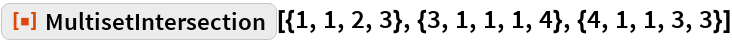Out=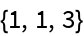Multisets as an Association of counts :

 In:=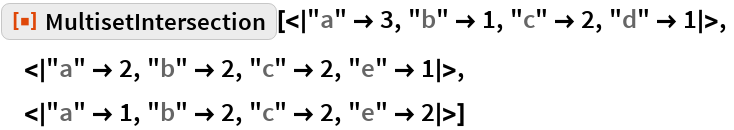Out=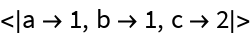### Scope

MultisetIntersection works with any head, not just List:

 In:=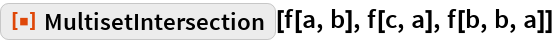Out=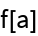### Properties and Relations

If there is no intersection, the result is {}:

 In:=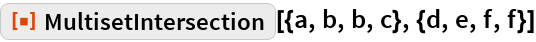Out=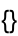If there is no intersection of the Association multisets, the result is <||>:

 In:=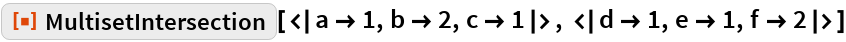Out=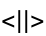Compare to Intersection:

 In:=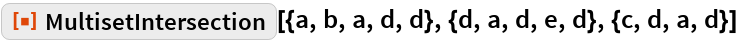Out=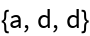In:=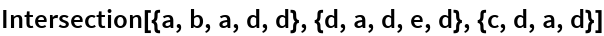Out=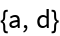## Requirements

Wolfram Language 11.3 (March 2018) or above